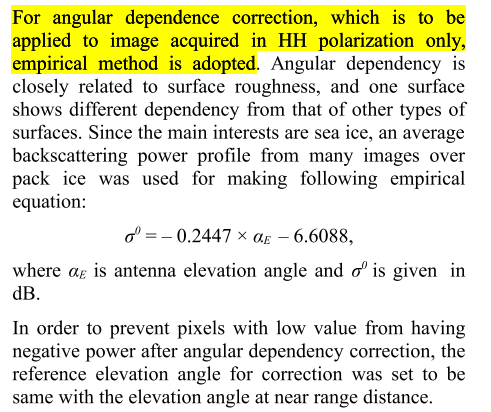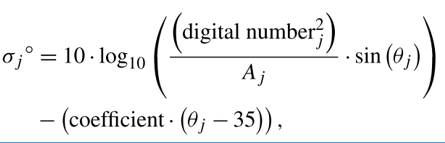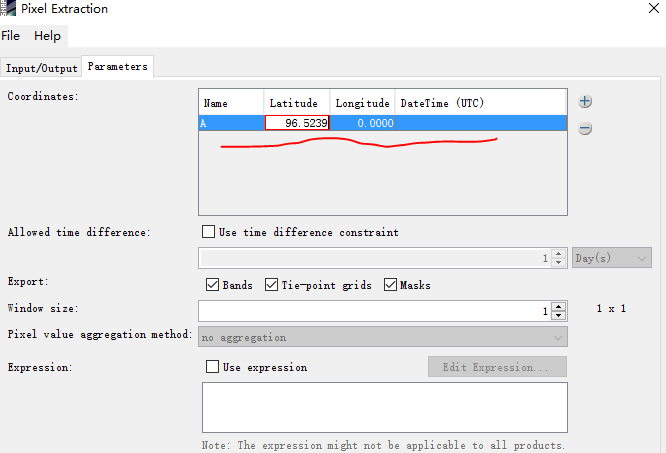# Backscatter normalization to a pre-defined incidence angle for S-1 GRD HH channel data

Hi, everyone.

I want to do backscatter normalization about the S-1 GRD HH channel data manually.Beside,I have found the formula below.

The introduction about the correction method is given in the next picture.However,I found the correction formula for Radarsat-2 HH channel data.The meaning of each symbol is in third picture.My question is whether the formula in picture 1 can be used to normalize the angular effect for Sentinel-1 HH channel data.
Besides, whether the equation in picture 1 is only the relationship between antenna elevation angle and backscatter coefficient sigma nought?

I would answer the second question first, be careful with the terms here elevation angle of the antenna could be something different than the incidence angle. Some platforms have the ability to move the SAR antenna and thus its pointing slightly, it is used mostly for calibration purposes. Incidence angle however is the range component of the beam, etc…definition wikipedia or smth. So here you would need to look into it, but I believe here the elevation angle is the incidence angle based on terrain/ice roughness.

Now for the usage of the RADARSAT formula, principally it should work yet you would need to set it up for coresponding Sentinel-1 values. The largest problem is to get the right coefficient for your Sigma0 function based on the incidence angle dependency, that is how you would go with a stable terrain. The idea is to calculate a representing Sigma0 function for each terrain element in your Area of Interest. Then you use this function to deliver a coefficient to compensate for the current measurement based on the range (incidence angle), thus returning normalized Sigma0 value.

Luckily if Range-Doppler Terrain corrected the Sigma0 values should be compensated enough for the range, thus a simple lieaner function should do the trick.

Hope this explanation helped a bit, otherwise this is what i am using for reference of my work:

Petr

1 Like

I see your meaning is to do the backscatter normalization using the Radarsat-2 formula by means of substituting responding Sentinel-1 values.

I still want to know whether the formula in picture 1 can be only used to get the normalized sigma0 value?If not,what’s the meaning of this formula?Or -0.2447 in picture 1 is the coefficient in formula of picture 2?

Thanks for your reply in advance.For the first formula I would assume that it is the coefficient like in the second picture just as you stated, only related to the described case scenario i. e. HH acquisitions of sea ice.

Plus they are trying to avoid values under the noise-equivalent sigma zero (NESZ), I don’t know the case scenario here, but for Sentinel-1 the value is -22[db]. (Page 59 https://sentinel.esa.int/documents/247904/349449/S1_SP-1322_1.pdf)

So yes it is principally similar approach as in the second formula only for the sea ice case and HH polarization, where you have to know for which satellite the values were meant.

Good Luck

I have made it ,thanks everyone.

1 Like

Thank you so much and your responses,I just did it and finished the backscatter normalization,now I wanna to know how to extract the backscatter coefficient of multiple area points for the study area.

You could after stacking or collocation, Use pins to export pixel values, Source of the postYeah,Idid that steps you recommended me ,which the tool “pixel extraction” didn’t work as shown in FIG.Could please tell me why ?

There is no latidude above 90°, you must enter the coordinates in geographic coordinates.

Source: https://en.wikipedia.org/wiki/File:Latitude_and_Longitude_of_the_Earth.svg

Here you can check for the correct coordinates:
https://www.sunearthtools.com/dp/tools/conversion.php?lang=en

First of all, thank you very much for your suggestion.I made it now.The reason for the problem is that I made a mistake in latitude and longitude.